## Find the number of solutions of the equation 6x^2+6x+3=0 by using the discriminant

Question

Find the number of solutions of the equation 6x^2+6x+3=0 by using the discriminant

in progress 0
5 months 2021-08-27T21:10:42+00:00 1 Answers 6 views 0

No real roots

Step-by-step explanation:

Given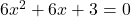Required

The number of solution

The discriminant (d) for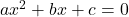is: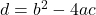So, we have: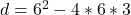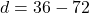Since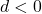, then the equation has no real roots